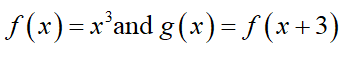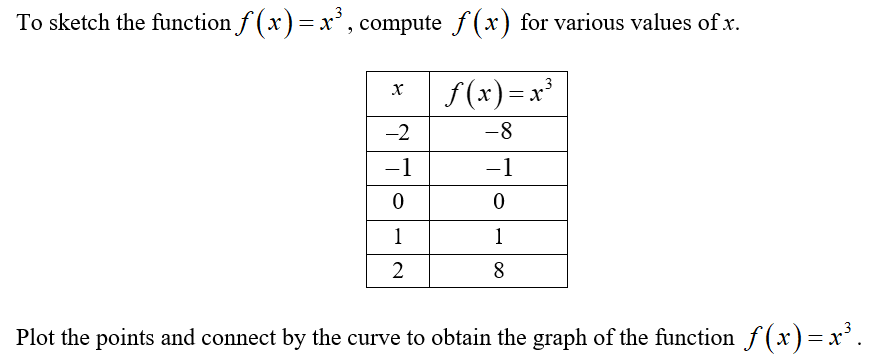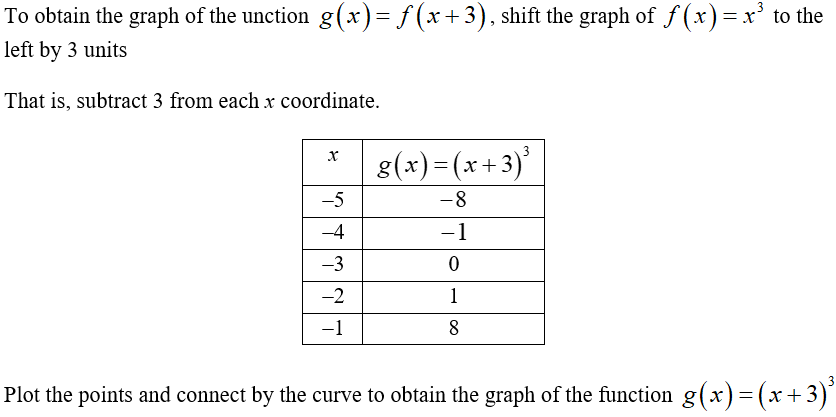# sketch the approximate shapes of the graph of the two functions on the same axes without using a calculator. Explain how the graphs of f(x) and g(x) are different from each other and how could you obtain the graph of g(x) from the graph of f(x). Find the points of intersection of the curves, if any.f(x)=x^3 and g(x)=f(x+3)

Question
1 views

sketch the approximate shapes of the graph of the two functions on the same axes without using a calculator. Explain how the graphs of f(x) and g(x) are different from each other and how could you obtain the graph of g(x) from the graph of f(x). Find the points of intersection of the curves, if any.

f(x)=x^3 and g(x)=f(x+3)

check_circle

The functions are given by,Sketch the graph of the function f(x).Sketch the graph of the function g(x)....

### Want to see the full answer?

See Solution

#### Want to see this answer and more?

Solutions are written by subject experts who are available 24/7. Questions are typically answered within 1 hour.*

See Solution
*Response times may vary by subject and question.
Tagged in